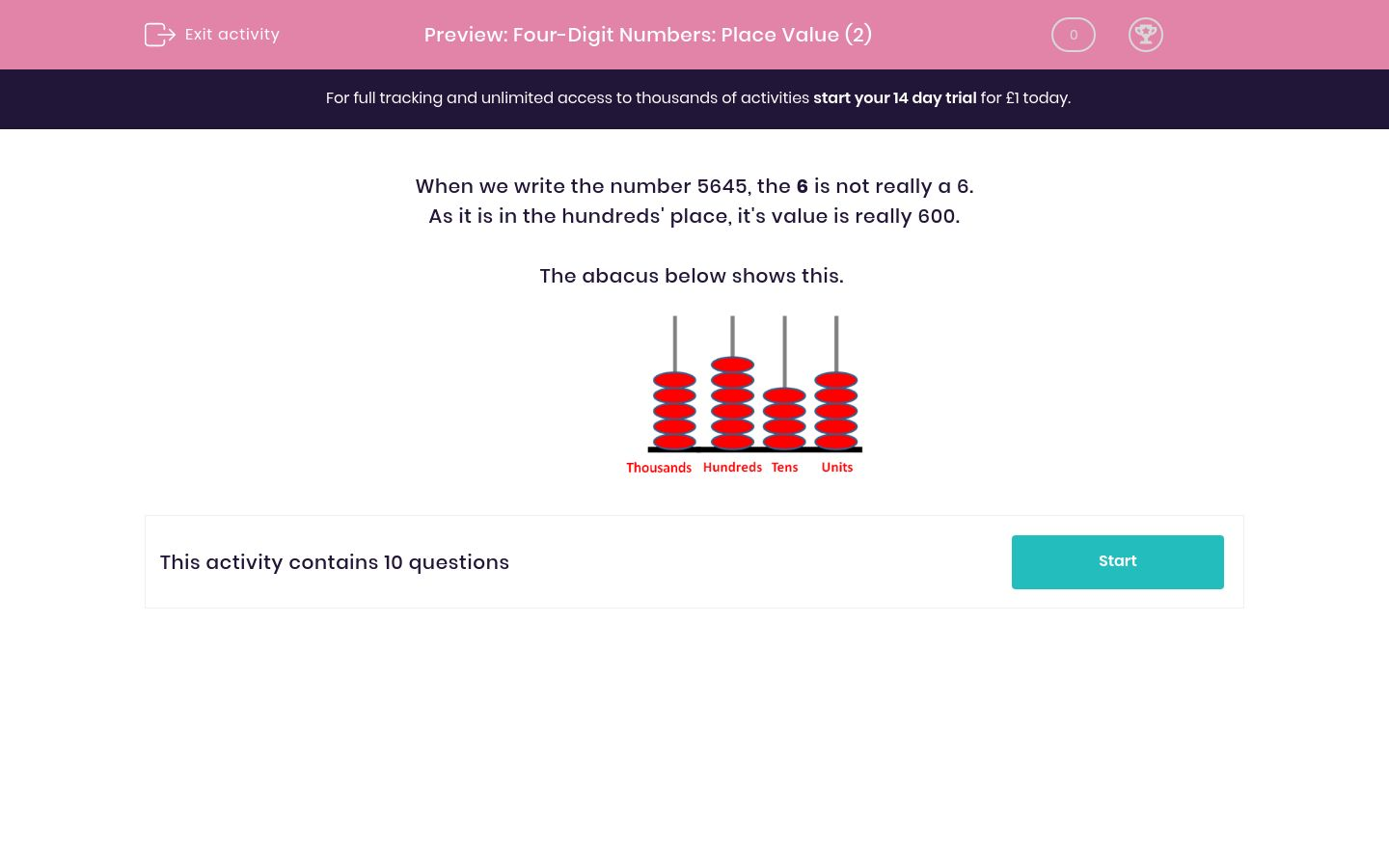# Four-Digit Numbers: Place Value (2)

In this worksheet, children must give the 'value' of a digit in a number.Key stage:  KS 2

Curriculum topic:   Maths and Numerical Reasoning

Curriculum subtopic:   Place Value

Difficulty level:### QUESTION 1 of 10

When we write the number 5645, the 6 is not really a 6.

As it is in the hundreds' place, it's value is really 600.

The abacus below shows this.What is the real value of the digit 5 in the number 2157 ?

5

50

500

5000

What is the real value of the digit 5 in the number 2527 ?

5

50

500

5000

What is the real value of the digit 5 in the number 5270 ?

5

50

500

5000

What is the real value of the digit 2 in the number 5270 ?

2

20

200

2000

What is the real value of the digit 2 in the number 5200 ?

2

20

200

2000

What is the real value of the digit 2 in the number 5012 ?

2

12

20

200

What is the real value of the digit 8 in the number 5018 ?

8

18

80

8000

What is the real value of the digit 1 in the number 1208 ?

1

10

100

1000

What is the real value of the digit 0 in the number 1208 ?

0

10

100

1000

What is the real value of the digit 0 in the number 1038 ?

0

10

100

1000

• Question 1

What is the real value of the digit 5 in the number 2157 ?

50
• Question 2

What is the real value of the digit 5 in the number 2527 ?

500
• Question 3

What is the real value of the digit 5 in the number 5270 ?

5000
• Question 4

What is the real value of the digit 2 in the number 5270 ?

200
• Question 5

What is the real value of the digit 2 in the number 5200 ?

200
• Question 6

What is the real value of the digit 2 in the number 5012 ?

2
• Question 7

What is the real value of the digit 8 in the number 5018 ?

8
• Question 8

What is the real value of the digit 1 in the number 1208 ?

1000
• Question 9

What is the real value of the digit 0 in the number 1208 ?

0
• Question 10

What is the real value of the digit 0 in the number 1038 ?

0
---- OR ----

Sign up for a £1 trial so you can track and measure your child's progress on this activity.

### What is EdPlace?

We're your National Curriculum aligned online education content provider helping each child succeed in English, maths and science from year 1 to GCSE. With an EdPlace account you’ll be able to track and measure progress, helping each child achieve their best. We build confidence and attainment by personalising each child’s learning at a level that suits them.

Get started